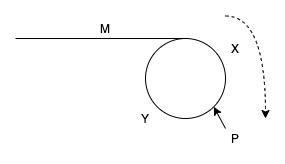# 快慢指针找环起点

28 Oct 2020 - algorithm

Given a linked list, return the node where the cycle begins. If there is no cycle, return null.
There is a cycle in a linked list if there is some node in the list that can be reached again by continuously following the next pointer. Internally, pos is used to denote the index of the node that tail’s next pointer is connected to. Note that pos is not passed as a parameter.
Notice that you should not modify the linked list.## 推理过程

$S_{fast} = 2 * S_{slow}$

$M + N * (Y + Y) + X = 2 * (M + X)$

$N * (Y + Y) = M$ $(N - 1) * (X + Y) + (X + Y) = M + X$ $(N - 1) * (X + Y) + Y = M$

$Y = M$

public class Solution {
return null;
}
while (fast != null && fast.next != null) {
fast = fast.next.next;
slow = slow.next;
if (fast == slow) {
break;
}
}
if (fast == null || fast.next == null) {
return null;
}